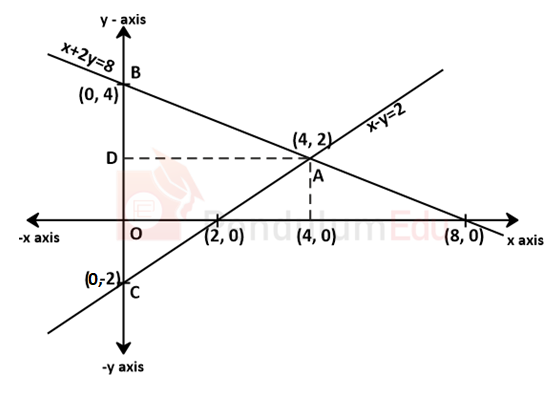Question of The Day01-11-2021

Find the area of the triangle formed by the straight line x – y = 2 , x + 2y = 8 and y- axis.

Correct Answer : a ) 12 sq. un.

Explanation :

According to the question

Straight lines are x – y = 2 ……..(1)

x + 2y = 8 …….(2)

x = 0 ……..(3)

Firstly, we need to find the points at which lines intersect, as these points will be the vertices of the triangle.

Subtracting eq(1) form eq (2)

⇒ 3y = 6

⇒ y = 2

⇒ x – y = 2

⇒ x = 2 + 2

⇒ x = 4

⇒ lines 1 and 2 intersect at A (4, 2)

⇒ Lines 1 and 3 intersect at C (0, -2)

⇒ Lines 2 and 3 intersect at B (0, 4)As shown in figure

Area of △ABC

Base (BC) = 4 + 2

⇒ 6 units

Area of triangle=$${1 \over 2}$$*base*height

$${1 \over 2}*6*4$$

⇒ 12 sq. units

Hence, (a) is the correct answer.0